Courses

# Inteference MCQ Level -2

## 10 Questions MCQ Test Topic wise Tests for IIT JAM Physics | Inteference MCQ Level -2

Description
This mock test of Inteference MCQ Level -2 for Physics helps you for every Physics entrance exam. This contains 10 Multiple Choice Questions for Physics Inteference MCQ Level -2 (mcq) to study with solutions a complete question bank. The solved questions answers in this Inteference MCQ Level -2 quiz give you a good mix of easy questions and tough questions. Physics students definitely take this Inteference MCQ Level -2 exercise for a better result in the exam. You can find other Inteference MCQ Level -2 extra questions, long questions & short questions for Physics on EduRev as well by searching above.
QUESTION: 1

### Two coherent monochromatic light beams of intensities l and 4I are superposed the maximum and minimum possible intensities in the resulting beam are :

Solution: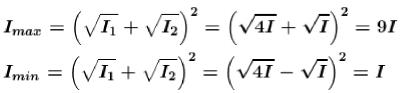The correct answer is: 9l and l

QUESTION: 2

### White light is used to illuminate the two slits in a young’s double slit experiment. The separation between the slit is b and the screen is at a distance d (>> b ) from the slits. At a point on the screen directly in front of one of the slits, certain wavelengths are missing. Some of these missing wavelengths are :

Solution:

Clearly at Q, path difference = 5 sin θ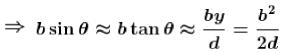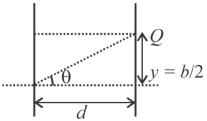Now whenever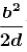will be odd multiple of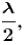,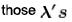will be having minima at point Q.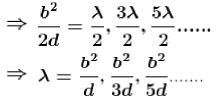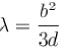QUESTION: 3

### The distance of nth bright fringe to the (n + 1) dark fringe in Young’s experiment is equal to :

Solution:

The distance between nth bright fringe and ( n + 1)m dark fringe is equal to half fringe width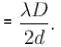The correct answer is: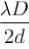QUESTION: 4

A light of frequency 5 x 1014 Hz enters a medium of refractive index 1.5. In this medium, the velocity of light wave is.

Solution: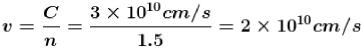The correct answer is: 2 x 1010  cm/sec

QUESTION: 5

Ratio of intensities of two light waves is given by 4 :1. The ratio of the amplitudes of the waves is :

Solution: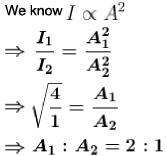The correct answer is: 2 : 1

QUESTION: 6

In a Fresnel biprism experiment, the two positions of lens given separation between the slits as 16 cm and 9 cm respectively. What is the actual distance of separation?

Solution:

The correct answer is: 12 cm

QUESTION: 7

A thin oil film of refractive index 1.2 floats on the surface of water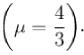When a light of wavelength λ = 9.6 x 10-7 m falls normally on the film from air, then it appears dark when seen normally. The minimum change in its thickness for which it will appear bright in normally reflected light by the same light is :

Solution:

For normal incidence path difference between ray 1 and ray 2 is 2μ1t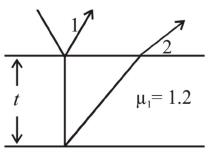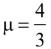For minimum thickness increment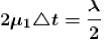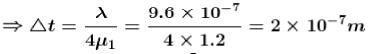The correct answer is: 2 x 10-7

QUESTION: 8

A parallel beam of monochromatic light is used in a Young’s double slit experiment. The slits are separated by a distance d and the screen is placed parallel to the plane of the slits. If the incident beam makes an angle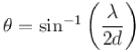with the normal to the plane of the slits, find the intensity of the geometrical centre p0.

Solution:

Clearly at 0, no difference of path due to inside motion of rays.
Only path difference = S1,Q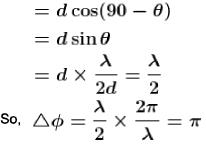⇒ destructive interference and hence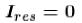QUESTION: 9

If one of the slits of a standard Young’s double slit experiment is covered by a thin parallel sided glass slab so that it transmits only one half the light intensity of the other, then :

Solution: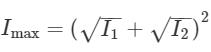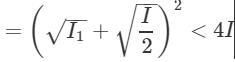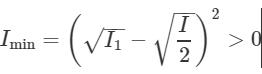QUESTION: 10

A young’s double slit experiment is performed with white light:

Solution:

The correct answer is: There will not be a completely dark fringe